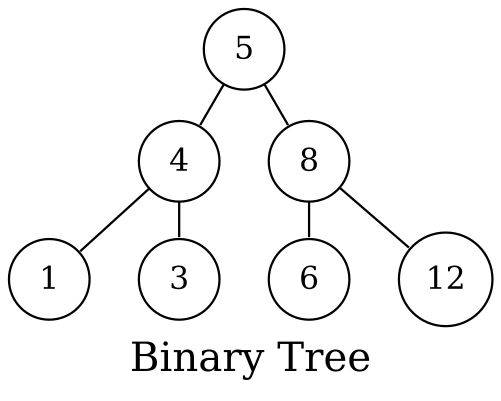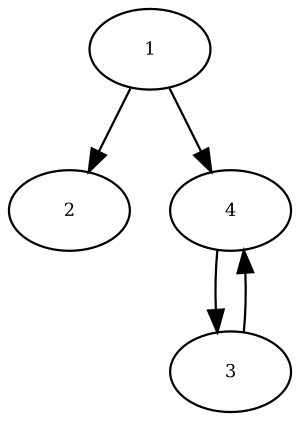# Practical Graph Theory in Ruby

This is the next installment in the “Practical Computer Science” series, where you will learn how to apply classic computer science concepts to solve real problems using Ruby.

Today we are going to talk about Graph Theory.

You may have heard about binary trees, they look like this:The thing is that a binary tree is just a specialized version of a graph, so that should give you an idea of how widespread graphs are.

Let’s start with an overview of graph theory fundamentals, then we are going to see what are some practical uses & how to implement this in Ruby!

## Graph Fundamentals

A graph is composed of two elements:

• Nodes (or vertices)
• Edges

One node represents one element in the graph, like a city or a street, in a graph representing a map. While the edges represent the connections between the nodes.

If you look at a computer science or math book you will see a graph defined by this formula: `G(V, E)`.

Where `G` means Graph, `V` is the set of vertices & `E` is the set of edges.

Graphs can be directed or undirected. Meaning that you can only go one direction (directed graph) or both directions (undirected graph).

The most popular type of graph is the Directed Acyclic Graph (DAG). Acyclic means that there are no loops, there is no way to backtrack.

## Uses for Graphs

Now that we have seen an overview of the fundamentals, let’s see some common uses for graphs.

Using graphs you can do things like:

• Find the shortest (or longest) path between two locations
• Check if two things are related to each other
• Build a recommendation engine
• Analyze dependencies

Another example includes finding the best route to a destination (think GPS devices).

## How to Implement & Use Graphs

You could write your own graph implementation, but for this article we are going to stick to the RGL gem which already implements one for us.

To create a basic graph using RGL:

```require 'rgl/adjacency'

```

This code produces the following graph:You can get a graphical representation of your graph like this:

```require 'rgl/dot'

graph.print_dotted_on
```

Then copy the output of that method on a site that can process the dot language. Like this one.

Alternatively, you can install Graphviz on your machine to produce the image locally.

Now that we have a graph, we may want to traverse it to find out information about it.

There are two basic algorithms for searching your graph:

• Depth-First Search (DFS)

In BFS you get the closest nodes first & in DFS you go as deep as possible for every node. These algorithms can be implemented using the stack data structure.

The RGL gem already implements those algorithms for you:

```require 'rgl/traversal'

graph.bfs_iterator.to_a
# [1, 2, 4, 3]

graph.dfs_iterator.to_a
# [1, 4, 3, 2]
```

Look at the graph again & follow the path these algorithms did using just your eyes (or you can use a finger too if you want). That will help you get a sense of what’s going on.

## Weighted Graphs

We can add more information to a graph in the form of weights to make it more useful.

Weights are given to edges, which are the paths between two nodes (also known as “vertices”). These weights represent the cost of going from one point to another.

For example, if we have a map of a country in the form of a graph & we want to reach a certain destination in the shortest time possible, the weights would represent the distance between two cities.Or if we have a computer network, the weights may represent how many hops it takes to reach a certain network.

“In computer networking, a hop is one portion of the path between source and destination. Data packets pass through bridges, routers and gateways as they travel between source and destination. Each time packets are passed to the next network device, a hop occurs.” – Wikipedia

Here’s a code example for a weighted graph:

```graph = RGL::DirectedAdjacencyGraph.new
graph.add_vertices "Los Angeles", "New York", "Chicago", "Houston", "Seattle"

edge_weights =
{
["New York", "Los Angeles"] => 2445,
["Los Angeles", "Chicago"] => 2015,
["Los Angeles", "Houston"] => 1547,
["Chicago", "Houston"] => 939,
["Seattle", "Los Angeles"] => 1548
}

edge_weights.each { |(city1, city2), w| graph.add_edge(city1, city2) }
```

We can now search for the shortest path from one point to another. And that’s exactly the topic of the next section!

## Finding The Shortest Path

A popular algorithm for finding the shortest path inside a graph is “Dijkstra’s Shortest Path” algorithm.

Given a weighted graph, we can use Dijkstra’s algorithm to solve this question:

“What’s the fastest way to get from point A to point B?”

Here is a code example, using the RGL gem:

```p graph.dijkstra_shortest_path(edge_weights, "New York", "Houston")
# ["New York", "Los Angeles", "Houston"]
```

This tells us the shortest path from New York to Houston, using the information available in the graph.

## Summary

You have learned what a graph data structure is & how to use it with the RGL gem.

You have also learned about common algorithms for working with graphs, like DFS, BFS & Dijkstra’s.

Don’t forget to share this post if you found it useful so more people can enjoy it 🙂

OmarPS says 3 years ago

Thanks for your post, I struggle a little bit to test the examples because of the requires, but the concepts are very clear.

Jesus Castello says 3 years ago

cs says 3 years ago

Liked the post you have done on rgl, fun stuff. I can’t figure out why I can’t get the dijkstra method call to work, maybe you have an idea? I have “rgl (0.5.3)” and I get everything else working by copying your examples.
The error: undefined method `dijkstra_shortest_path’ for # (NoMethodError)

Jesus Castello says 3 years ago

Make sure to require `rgl/traversal`.

cs says 3 years ago

I have that dependency and also ‘require ‘rgl/adjacency’. Strange one, because I got graph.add_vertices and graph.add_edge to work but not graph.dijkstra_shortest_path – still no method error. I’m stumped.

Luke says 3 years ago

I think someone is stealing your blog posts — I didn’t see anything pointing to you or that you wrote it at least. It looks like they took full credit.

Jesus Castello says 3 years ago

Thanks for letting me know. I didn’t write that or give permission to republish.

Greg says 3 years ago

Thanks for share. I think need to add：require ‘rgl/traversal’

Jesus Castello says 3 years ago

Yes, you do. Thanks for reading 🙂